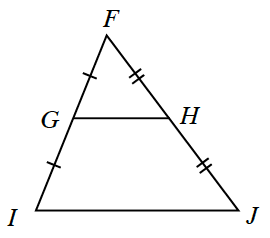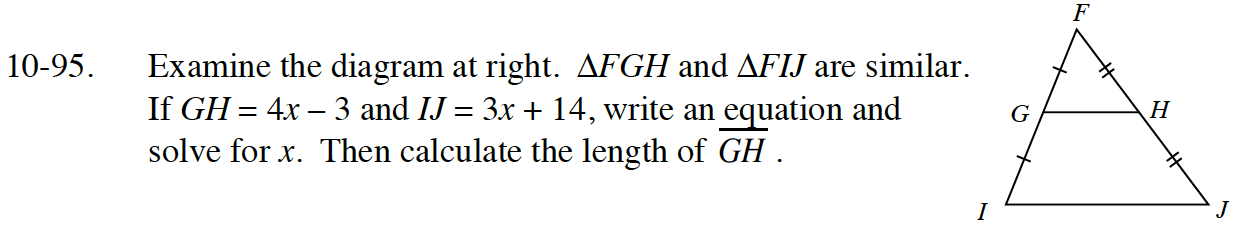### Home > CC4 > Chapter 10 > Lesson 10.2.2 > Problem10-95

10-95.Examine the diagram at right. ΔFGH and ΔFIJ are similar. If GH = 4x – 3 and IJ = 3x + 14, write an equation and solve for x. Then calculate the length of GH. Homework Help ✎Since G is the midpoint of segment FI and H is the midpoint of segment FJ, segment IJ is twice as long as GH.